# The farmer

The farmer had 140 sheep. For the next year, she decided to change the number of sheep in ratio 10: 7. How many sheep will he have then?

n =  98

### Step-by-step explanation:

140 = 10/7 • n

10n = 980

n = 98

Our simple equation calculator calculates it.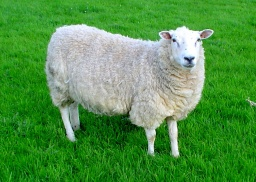Did you find an error or inaccuracy? Feel free to write us. Thank you!Tips to related online calculators
Need help to calculate sum, simplify or multiply fractions? Try our fraction calculator.
Check out our ratio calculator.
Do you have a linear equation or system of equations and looking for its solution? Or do you have a quadratic equation?

## Related math problems and questions:

• Magic bagEach time the prince crossed the bridge, the number of tolars in the magic bag doubled. But then the devil always conjured 300 tolars for him. When this happened for the third time, the prince had twice as much as he had in the beginning. How many tolars
• The farmerThe farmer calculated that the supply of fodder for his 20 cows was enough for 60 days. He decided to sell 2 cows and a third of the feed. How long will the feed for the rest of the peasant's herd last?
• Apples investment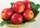The merchant bought a certain amount of apples and paid 1 denarius for every 7 apples. The next day he sold all the apples for 1 denarius for 5 pieces and earned 12 denarii. How many apples did he originally buy and how many did he have to invest in this
• Cottages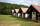The summer camp is 41 cottages. Rooms are for three and four in them. How many of the 140 campers lives of three?
• Fewer than 500 sheep,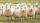There are fewer than 500 sheep, but if they stand in a double, triple, quadruple, five, and sixth-order, one sheep will remain. But they can stand in the seventh order. How many are sheep?
• The farmer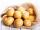The farmer brought potatoes to the market. In the first hour he sold two-fifths of the potatoes brought in, in the second hour he sold five-sixths of the remaining potatoes, and in the third hour, he sold the last 40 kg of potatoes. 1. Express a fraction
• Bricklayers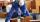8 bricklayers build a house for 630 days. How many bricklayers have to add after 150 days to complete the whole building in (next) 320 days?
• Cinema 5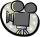James wants to go to the movies every Tuesday for five years. After a year he learned that the ticket price on Wednesday is only € 5.10 and € 5.60 the other day. Now that he know about the discount, he decided to change his habits and he will go to the ci
• Unknown numberSamuel wrote unknown number. Then he had add 200000 to the number and the result multiply by three. When it calculated he was surprised, because the result would have received anyway, if write digit to the end of original number. Find unknown number.
• Two numbersWe have two numbers. Their sum is 140. One-fifth of the first number is equal to half the second number. Determine those unknown numbers.
• The farmer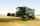The farmer harvested 840 tons of grain in 2006, which was 44% less than in 2005, and one-fifth more than in 2004. How many tons of grain harvested in years 2005 and 2004?
• The balls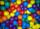You have 108 red and 180 green balls. You have to be grouped into the bags so that the ratio of red to green in each bag was the same. What smallest number of balls may be in one bag?
• Jan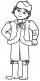Jan is 13 years and Peter 21 years old. For how many years will their ages in the ratio of 7:9?
• The inheritanceDad originally decided to bequeath the money to his two sons so that they would share it 8: 7 (older and younger). Then he changed his mind and divided the same amount in the ratio 11: 9 (older and younger). This increased the value of the inheritance for
• Change the numbers in the ratio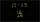Change the numbers 29, 38, and 43 in a 3: 4 ratio.
• UN 1If we add to an unknown number his quarter, we get 210. Identify unknown number.
• Rajendra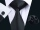Rajendra a farmer had two sons and two daughters. He decided to divide his property among his sons and daughters. So he wrote a "WILL" about distribution of his property. According to his "WILL", he desired to give 3/5 th of the property to his sons in eq